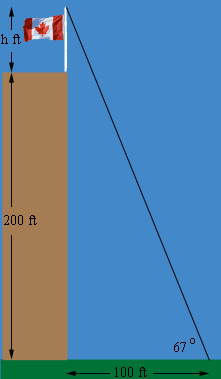SEARCH HOMEMath Central Quandaries & QueriesQuestion from Micah, a student: A flagpole stands vertically at the edge of a roof of a building 200 ft high. the angle of elevation of the pole from a point 100 ft from the bottom of the building is 67 degrees. Find the length of the pole in meters.Hi Micah,

I drew a diagram.I let h be the height of the flag pole. In the triangle in the diagram what trig function relates the height (200 + h ft) the base (100 ft) and the angle with measure 67 degrees? Use this trig function to find h.

PennyMath Central is supported by the University of Regina and The Pacific Institute for the Mathematical Sciences.